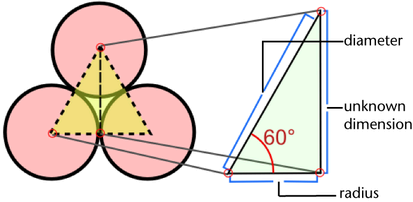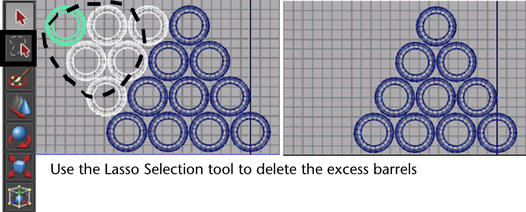Sine is the ratio obtained by dividing the side opposite the angle by the hypotenuse. Since the center points of the barrels are spaced along the points of an equilateral triangle, all angles of the triangle are equal to 60°. If you multiply the sine of 60° by the length of one of the sides of the triangle, the result is the height of the triangle.It is important to know which units of measurement the built-in functions of a programming language use. Many programming languages, including MEL and Python, perform trigonometric operations in radians. You must convert from degrees to radians when using the sine function to calculate the Y offset.

NoteRadians are a method of measuring sections of a circle. There are 360 degrees in a circle, which is equivalent to 2pi radians.

To calculate the offset in Y

1. Declare a new variable to store the value of the Y offset by typing the following:
`float \$Y_offset;`
2. Calculate the value of the Y-offset using the sine ratio. You must convert the value of the angle to degrees with the degree to radian conversion function.
` \$Y_offset = \$diameter_barrel * (sin(deg_to_rad(60)));`

The assignment operator evaluates the right hand side of the expression and assigns it to the left hand side. The degree to radian conversion function is the first to be evaluated, as it is within the most interior set of brackets. The sine function calculates the sine of the angle given in radians. The sine value is multiplied by the diameter to calculate the Y offset. The variable is assigned the calculated value.

NoteThe variable \$diameter_barrel does not change in value. Only the variable on the left side of an assignment operator changes in value.

To stack the rows of barrels

1. Select and duplicate all the objects in the scene, by typing the following:
`select -allDagObjects;`
`duplicate;`

Using the select command with the -allDagObjects flag selects all DAG (Directed Acyclic Graph) objects, which are a set of objects that exist in the scene such as geometry, IK chains and measure tools. Textures and animation information do not belong to the Directed Acyclic Graph.

2. Offset the barrels by the radius of a barrel in X and the calculated \$Y_offset in Y, divide the diameter by 2 to get the value of the radius. When duplicating the rows, you have created too many barrels.
`move -r 0 \$Y_offset (\$diameter_barrel/2.0);`
`duplicate;`
`move -r 0 \$Y_offset (\$diameter_barrel/2.0);`
`duplicate;`
`move -r 0 \$Y_offset (\$diameter_barrel/2.0);`
3. Using the Lasso tool, select the extra barrels.
4. Delete the extra barrels to obtain a pyramid shape.